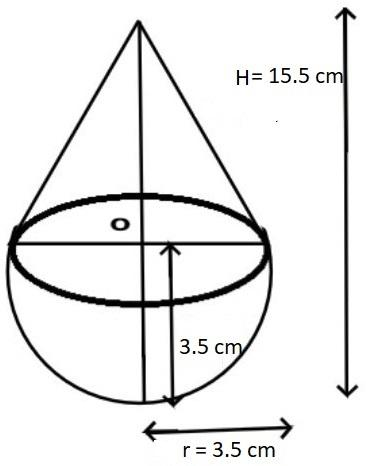A toy is in the form of a cone mounted on a hemisphere of radius 3.5 cm. The total height of the toy is 15.5 cm. find the total surface area and the volume of the toy.Verified
79.6k+ views
Hint: In this particular question first draw the pictorial representation it will give us a clear picture of what we have to find out then use the concept that the total surface area of the toy is the sum of the lateral surface area of the cone and the curved surface area of the hemisphere, so use these concepts to reach the solution of the question.Given data:
Height of the toy = 15.5 cm.
Radius of the hemisphere = 3.5 cm, as shown in the above figure.
From the figure we can say that the radius of cone = radius of the hemisphere = 3.5 cm
Height of the cone (h) = height of the toy – radius of the hemisphere.
So, the height of the cone (h) = 15.5 – 3.5 = 12 cm.
Now as we know that the slant height of the cone is given as
Slant height (l) = $\sqrt {{{\left( {{\text{height of the cone}}} \right)}^2} + {{\left( {{\text{radius of the cone}}} \right)}^2}}$
Now substitute the values we have,
Slant height (l) = $\sqrt {{{\left( {{\text{12}}} \right)}^2} + {{\left( {{\text{3}}{\text{.5}}} \right)}^2}} = \sqrt {144 + 12.25} = \sqrt {156.25} = 12.5$cm.
Now as we know that the lateral surface area (LSA) of the cone is given as,
LSA = $\pi rl$ square units, where r and l are the radius and the slant height of the cone.
$\Rightarrow {\text{LSA}} = \dfrac{{22}}{7}\left( {3.5} \right)\left( {12.5} \right) = 137.5$ ${cm}^2$.
Now as we know that the curved surface area (CSA) of the hemisphere is $2\pi {r^2}$ where r is the radius of the hemisphere.
Therefore, CSA = $2\left( {\dfrac{{22}}{7}} \right){\left( {3.5} \right)^2} = 77$ ${cm}^2$.
So, the total surface area of the toy is the sum of the lateral surface area of the cone and the curved surface area of the hemisphere.
Therefore, the total surface area (TSA) of the toy = LSA of the cone + CSA of the hemisphere.
Therefore, the total surface area (TSA) of the toy = 137.5 + 77 = 214.5 ${cm}^2$.
Now as we know that the volume of the cone = $\dfrac{1}{3}\pi {r^2}h$ cubic units, where r and h are the radius and the height of the cone.
And the volume of the hemisphere = $\dfrac{2}{3}\pi {r^3}$ cubic cm, where r is the radius of the hemisphere.
So the total volume of the toy = volume of the cone + volume of the hemisphere.
So the total volume of the toy = $\dfrac{1}{3}\pi {r^2}h$ + $\dfrac{2}{3}\pi {r^3}$ ${cm}^3$.
Now substitute the values we have,
So the total volume of the toy is,
$\Rightarrow \dfrac{1}{3}\left( {\dfrac{{22}}{7}} \right){\left( {3.5} \right)^2}\left( {12} \right) + \dfrac{2}{3}\left( {\dfrac{{22}}{7}} \right){\left( {3.5} \right)^3} = 154 + 89.833 = 243.83$ ${cm}^3$.

So this is the required answer.
Note: Whenever we face such types of questions the key concept we have to remember is that always recall the formula of the lateral surface area of the cone and the curved surface area of the hemisphere which is all stated above and also recall the volume of the cone and the volume of the hemisphere which is also stated above.